# Plus One Maths Chapter Wise Questions and Answers Chapter 12 Introduction to Three Dimensional Geometry

Students can Download Chapter 12 Introduction to Three Dimensional Geometry Questions and Answers, Plus One Maths Chapter Wise Questions and Answers helps you to revise the complete Kerala State Syllabus and score more marks in your examinations.

## Kerala Plus One Maths Chapter Wise Questions and Answers Chapter 12 Introduction to Three Dimensional Geometry

### Plus One Maths Introduction to Three Dimensional Geometry Three Mark Questions and Answers

Question 1.
Prove by using distance formula that the A(1, 2, 3), B(-1, -1, -1) and C(3, 5, 7) are collinear.Now BC = AB + AC
Thus A, B, C are collinear.Question 2.
Verify the following: (3 score each)

1. (0, 7, -10), (1, 6, -6) and (4, 9, -6) are the vertices of an isosceles triangle.
2. (0, 7, 10), (-1, 6, 6) and (-4, 9, 6) are the vertices of a right angled triangle.
3. (-1, 2, 1), (1, -2, 5), (4, -7, 8) and (2, -3, 4) are the vertices of a parllelogram.

1. Let A(0, 7, -10), B(1, 6, -6) and C(4, 9, -6) be the two points.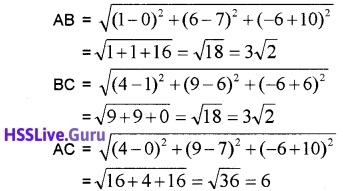Now AB = BC, thus ABC is an isosceles triangle.

2. Let A(0, 7, 10), B(-1, 6, 6) and C(-4, 9, 6) be the two points.Now AC2 = AB2 + BC2, thus ABC is a right triangle.

3. Let A(-1, 2, 1), B(1, -2, 5), C(4, -7, 8) and D(2, -3, 4) be the two points.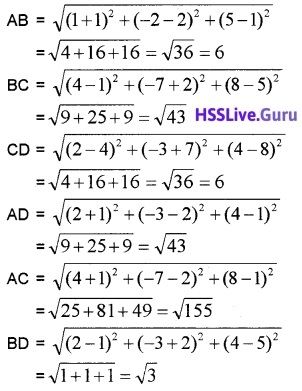Now AB = CD, BC = AD, AC ≠ BD, thus A, B, C, D are vertices of a parallelogram.Question 3.
Find the equation of set points which are equidistant from the points (1, 2, 3) and (3, 2, -1).
Let P(x,y,z) be any point which is equidistant from the point A(1, 2, 3) and B (3, 2, -1).
Given; PA = PB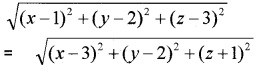(x – 1)2 + (y – 2)2 + (z – 3)2
= (x – 3)2 + (y – 2)2 + (z + 1)2
= x2 – 2x + 1 + y2 – 4y + 4 + z2 – 6z + 9
= x2 – 6x + 9 + y2 – 4y + 4 + z2 + 2z + 1 – 2x + 14 – 6z = -6x + 14 + 2z
⇒ 4x – 8z = 0
⇒ x – 2z = 0.

Question 4.
Find the coordinate of the point which divides the line segment joining the points (3, -2, 5) and (3, 4, 2) in the ratio 2:1 (3 score each)

1. 2:1 internally
2. 2:1 externally

1. Let P(x, y, z) be any point which divides the line segment joining points A(3, -2, 5) and B (3, 4, 2) in the ratio 2:1 internally.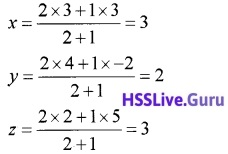Therefore coordinates of P are (3, 2, 3).

2. Let P(x, y, z) be any point which divides the line segment joining points A(3, -2, 5) and B (3, 4, 2) in the ratio 2:1 externally.Therefore coordinates of P are (3, 10, -1).Question 5.
Find the ratio in which the line joining the points (1, 2, 3) and (-3, 4, -5) is divided by the xy-plane.
Let the line joining the points A(1, 2, 3) and B(-3, 4, -5) is divided by the xy-plane in the ratio k:1.
Then the coordinateSince the point lies on xy-axis, we have;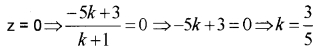Thus the required ratio is $$\frac{3}{5}$$; ie, 3:5.

Question 6.
Find the coordinates of the points which trisect the line segment joining the points P(4, 2, -6) and Q (10, -16, 6).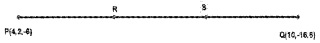Let R and S be two points which trisect the line join of PQ. Therefore PR = RS = SQ Then coordinate of R is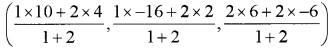= (6, -4, -2)
Then coordinate of S is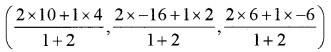= (8, -10, 2).

### Plus One Maths Introduction to Three Dimensional Geometry Practice Problems Questions and Answers

Question 1.
Find the distance between the following pair of points: (1 score each)

1. (2, 3, 5) and (4, 3, 1)
2. (-3, 7, 2) and (2, 4, -1)
3. (-1, 3, -4) and (1, -3, 4)